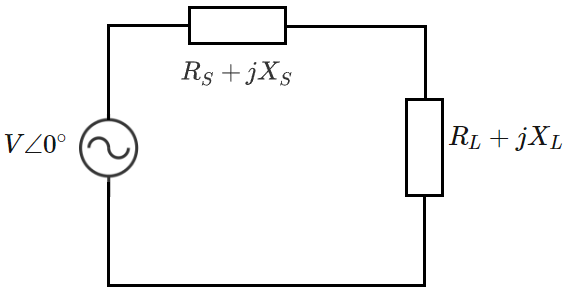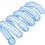# Conjugate Impedance MatchingConsider the AC system shown above. There is a source with impedance $R_S + jX_S$ and a load with impedance $R_L + jX_L$. The resistive $(R)$ components must be positive (per standard physics), and the reactive $(X)$ components can be either positive or negative (corresponding to inductive / capacitive components respectively). The source voltage angle is shown as zero degrees, but this angle is actually irrelevant.

Suppose we want to maximize the active power (Watts) dissipated by the load. What should the load impedance parameters be?

Derive an expression for the magnitude of the load current:

$|I| = \frac{V}{\sqrt{(R_S + R_L)^2 + (X_S + X_L)^2}} \\ |I|^2 = \frac{V^2}{(R_S + R_L)^2 + (X_S + X_L)^2}$

Load power:

$P_L = |I|^2 R_L = \frac{V^2 R_L}{(R_S + R_L)^2 + (X_S + X_L)^2}$

Since $V$ is a constant, we therefore want to maximize the following quantity:

$\alpha = \frac{R_L}{(R_S + R_L)^2 + (X_S + X_L)^2}$

Assume that this function is both continuous and continuously differentiable in both variables $(R_L, X_L)$. Take partial derivatives with respect to both variables and set them equal to zero. This is a multi-variate version of the standard calculus-based optimization strategy. I will also neglect to perform the equivalent of a "second derivative test" here. The mathematically inclined reader is free to try these as side problems. I don't want to get bogged down in mathematical details.

$\frac{\partial{\alpha}}{\partial{R_L}} = \frac{(R_S + R_L)^2 + (X_S + X_L)^2 - R_L (2(R_S + R_L))}{\beta} = 0 \\ \implies (R_S + R_L)^2 + (X_S + X_L)^2 = 2 R_L (R_S + R_L)$

$\frac{\partial{\alpha}}{\partial{X_L}} = \frac{-2 R_L (X_S + X_L)}{\beta} = 0 \\ \implies R_L (X_S + X_L) = 0$

Since we are maximizing the load active power, we must obviously neglect the case in which $R_L = 0$.

$\implies X_S + X_L = 0 \\ \boxed{X_L = -X_S}$

The resulting expression from the $R_L$ partial derivative thus reduces to:

$(R_S + R_L)^2 = 2 R_L (R_S + R_L)$

Since the resistive terms cannot be negative, and $R_L$ cannot be zero, the following results:

$R_S + R_L = 2 R_L \\ \boxed{R_L = R_S}$

We see, therefore, that in order to maximize the active power dissipated by the load, the load resistance must match the source resistance, and the load reactance must be the negative of the source reactance. In more technical language, the load impedance must be the complex conjugate of the source impedance.Note by Steven Chase
3 years, 10 months ago

This discussion board is a place to discuss our Daily Challenges and the math and science related to those challenges. Explanations are more than just a solution — they should explain the steps and thinking strategies that you used to obtain the solution. Comments should further the discussion of math and science.

When posting on Brilliant:

• Use the emojis to react to an explanation, whether you're congratulating a job well done , or just really confused .
• Ask specific questions about the challenge or the steps in somebody's explanation. Well-posed questions can add a lot to the discussion, but posting "I don't understand!" doesn't help anyone.
• Try to contribute something new to the discussion, whether it is an extension, generalization or other idea related to the challenge.
• Stay on topic — we're all here to learn more about math and science, not to hear about your favorite get-rich-quick scheme or current world events.

MarkdownAppears as
*italics* or _italics_ italics
**bold** or __bold__ bold
- bulleted- list
• bulleted
• list
1. numbered2. list
1. numbered
2. list
Note: you must add a full line of space before and after lists for them to show up correctly
paragraph 1paragraph 2

paragraph 1

paragraph 2

[example link](https://brilliant.org)example link
> This is a quote
This is a quote
    # I indented these lines
# 4 spaces, and now they show
# up as a code block.

print "hello world"
# I indented these lines
# 4 spaces, and now they show
# up as a code block.

print "hello world"
MathAppears as
Remember to wrap math in $$ ... $$ or $ ... $ to ensure proper formatting.
2 \times 3 $2 \times 3$
2^{34} $2^{34}$
a_{i-1} $a_{i-1}$
\frac{2}{3} $\frac{2}{3}$
\sqrt{2} $\sqrt{2}$
\sum_{i=1}^3 $\sum_{i=1}^3$
\sin \theta $\sin \theta$
\boxed{123} $\boxed{123}$

## Comments

There are no comments in this discussion.

×

Problem Loading...

Note Loading...

Set Loading...